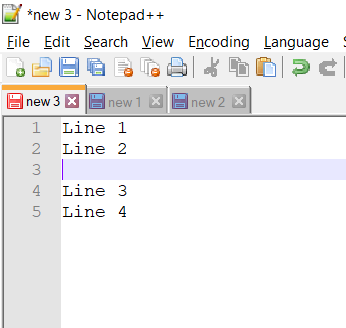# Notepad++ insert a blank line above or below the current line exampleIf you are a developer I am sure you would be looking for a way to enter a new blank line just above or below the line you are in Notepad++ Text editor, I looked for it and could not find any short cut so thought of creating a macro for it, you need to import it in your shortcuts.xml.

To add a blank line below the current line:
``````        <Macro name="New Line below" Ctrl="yes" Alt="yes" Shift="yes" Key="40">
<Action type="0" message="2451" wParam="0" lParam="0" sParam="" />
<Action type="1" message="2170" wParam="0" lParam="0" sParam="&#x000D;" />
<Action type="1" message="2170" wParam="0" lParam="0" sParam="&#x000A;" />
</Macro>``````

Press Ctrl + Alt + Shift + Down to add a new line just below the current line. The macro will go to the end of the line for the current line and press enter!

To add a blank line above the current line:
``````        <Macro name="Add Line above" Ctrl="yes" Alt="yes" Shift="yes" Key="38">
<Action type="0" message="2451" wParam="0" lParam="0" sParam="" />
<Action type="0" message="2302" wParam="0" lParam="0" sParam="" />
<Action type="1" message="2170" wParam="0" lParam="0" sParam="&#x000D;" />
<Action type="1" message="2170" wParam="0" lParam="0" sParam="&#x000A;" />
</Macro>``````

Press Ctrl + Alt + Shift + Down to add a new line just below the current line.